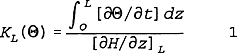# Abstracts

It is theoretically shown that unit hydraulic potential gradients cannot occur in homogeneous soils undegoing internal drainage process even though this assumption has been used successfully by several authors of soil hydraulic conductivity methods.

soil hydraulic conductivity; unit gradient; internal drainage

É mostrado teoricamente que gradientes unitários de potencial hidráulico da água não podem ocorrer em perfis homogêneos de solo sob drenagem interna, apesar desta aproximação ter sido utilizada com sucesso em vários métodos de determinação de condutividade hidráulica do solo.

NOTES

Unit gradient in internal drainage experiments for the determination of soil hydraulic conductivity

Gradiente unitário em experimentos de drenagem interna para determinação da condutividade hidráulica do solo

K. Reichardt

Prof. of Dep. of Physics and Meteorology, University of São Paulo, ESALQ, C.P. 9 - CEP:13418-900-Piracicaba,SP

ABSTRACT

It is theoretically shown that unit hydraulic potential gradients cannot occur in homogeneous soils undegoing internal drainage process even though this assumption has been used successfully by several authors of soil hydraulic conductivity methods.

Key Words: soil hydraulic conductivity, unit gradient, internal drainage.

RESUMO

É mostrado teoricamente que gradientes unitários de potencial hidráulico da água não podem ocorrer em perfis homogêneos de solo sob drenagem interna, apesar desta aproximação ter sido utilizada com sucesso em vários métodos de determinação de condutividade hidráulica do solo.

Many methods used for the determination of unsaturated soil hydraulic conductivity are based on experimental designs involving the drainage of previously saturated soil profiles Preventing soil surface evaporation and assuming water flow occurring only in the vertical direction, the integration of Richard's equation yields the basic equation for the estimation of unsaturated hydraulic conductivity KL(q):where:

q = volumetric soil water content (cm3cm-3).

H = total hydraulic pressure head (cm),assumed as the sum of matric pressure head h (cm) and gravitational pressure head z (cm).

t = time (s).

z = vertical space coordinate (cm), L being a particular value of z at which K(q) is determined.

Since the integral in equation (1) strongly determines the value of K(q) and since many experiments indicate that the hydraulic gradient is close to 1 due to the dominance of gravity, and because of the extra efforts to be devoted to measureH/z, several authors use the so called "unit gradient assumption". This assumption was first introduced by DAVIDSON et al., (1969) and then used in several field methods (e.g. CHONG et al., 1979; LIBARDI et al., 1980, SISSON et al., 1980). The validity of this assumption is frequently questioned; one clear example has been published by AHUJA et al (1988).

Although gradients close to unit are frequently observed during internal drainage, it is my point of view that theoretically unit gradients cannot occur, even for a homogeneous soil profile. Assuming H = h + z, the conditionH/z = 1 implies inh/z = 0, and as a consequence h = constant in depth, for all t. The soil being homogeneous, there is a unique h(q) relation and, as a result, q will also be constant in depth.(Figure 1) ilustrates these points. The constant q profiles shown in figure 1C indicate that K(q) calculated according equation (1) (consideringH/z = 1, as assumed) must increase in depth:which contradicts the first assumption of an homogeneous soil. Therefore, cannot be zero, and should actually decrease during drainage time until equilibrium is reached, when= 0, and water flow stops.

Figure 2 ilustrates this point. At time tf the soil reaches the theoretical soil water equilibrium, when the Darcian flux density (cm.s-1) becomes zero (H/z =1-1=0) and matric forces counteract gravity. In fact q becomes very close to zero much before tf, due to the exponential character of the K(q) function.

In practice, it is frequently observed that soil profiles present fairly parallel water content profiles during the evolution of the internal drainage process and, as a consequence K, values for given q values will increase in depth.

Does this reflect the reality or is it an artifact of our calculations? I wonder if K values would decrease in depth if those soil profiles could be inverted.

Trabalho entregue para publicação em 08.04.92

Trabalho aprovado para publicação em 24.06.92

• AHUJA, L.R.; BARNES, B.B.; KASSEL, D.K.; BRUCE, R.R. & NOFZIGER, D.L., (1988): Effect of assumed unit gradient during drainage on the determination of unsaturated hydraulic conductivity and infiltration parameters. Soil Science, v.145, p.235-243.
• CHONG, S.R.; GREEN, R.E. & AHUJA, L.R. (1979): Simple in situ determination of hydraulic conductivity by power function descriptions of drainage. Water Resources Research, v.17, p.1109-1114.
• DAVIDSON, J.M.; STONE, L.R.; NIELSEN, D.R. & LARUE, M.E. (1969). Field measurement and use of soil-water properties. Water Resour. Research, v.5, p.1312-1321.
• LIBARDI, P.L.; REICHARDT, K.; NIELSEN, D.R. & BIGGAR, J.W. (1980): Simple field methods for estimating soil hydraulic conductivity. Soil Science Society of American Journal, v.44, p.3-7.
• SISSON, J.B.; FERGUSON, A.H. & VAN GENUCHTEN, M.Th. (1980): Simple method for predicting drainage from field plot s. Soil Science Society of American Journal, v.44, p. 1147-1152.

# Publication Dates

• Publication in this collection
02 Aug 2005
• Date of issue
May 1993

• Accepted
24 June 1992